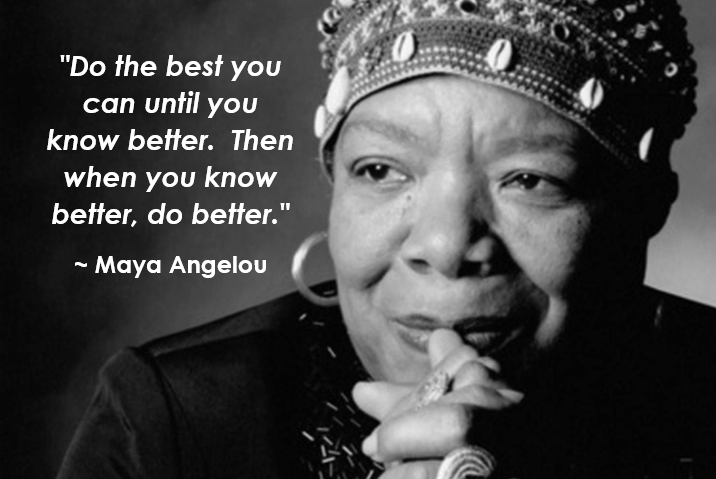Strategies are listed from the earliest strategies up through the standard algorithm.  Many are used side by side, but it is important to understand that the variety of strategies are used to build a deeper conceptual understanding and move to a more procedural model backed by conceptual understanding of addition.  Keep in mind that mastery of the standard algorithm of addition is not expected until grade 4 per the Maine Learning Results and Common Core State Standards, however students will begin practicing the standard algorithm along side other strategies much earlier than grade 4.

#####Students begin their understanding of addition by modeling out the actions in a joining situation.  They may draw pictures, use counters or cubes, use their fingers, or even use five or ten frames.  Students will need exposure to different types of addition problems such as add to, put together, or compare problems where they could be looking to find either a sum or a missing addend in the problem.  Students also need practice with equations where the missing sum or addend is on the left side of the equal symbol as opposed to the typical sum to the right of the equal symbol.  The equal symbol does not signify "the answer is" but rather the expressions on either side of the equal symbol represent the same value.  For more examples of addition problem situations, check out the Glossary Table 1 from the Common Core State Standards for Mathematics.

One major concept that students work on early in addition is making combinations of 10.  As students learn these combinations, it strengthens their ability to compose (put together) or decompose (take apart) numbers.  This in turn helps with their fluency and building their tool box of more efficient strategies.

##### Counting Up & Counting On

What is the difference between the Counting Up and Counting On strategies?  The Counting Up strategy is when students count all the numbers as they are adding.  For example, when adding three plus two, they would say "one, two, three...four, five".  The Counting On strategy is when students hold on to one number and continue counting on the number added.  For example,  when doing the same problem, adding three plus two, the student would say "three...four, five".  Students typically start with Counting Up and gradually move on to Counting On when they can hold one value as a group - typically the larger value.

##### Number Grids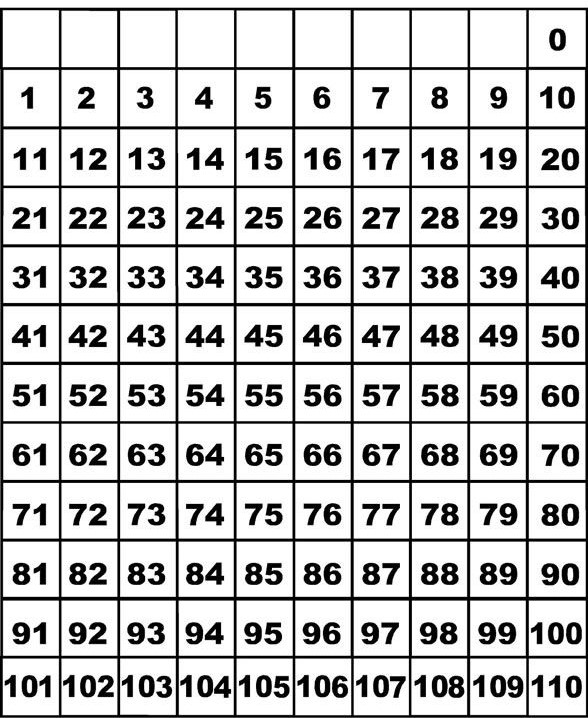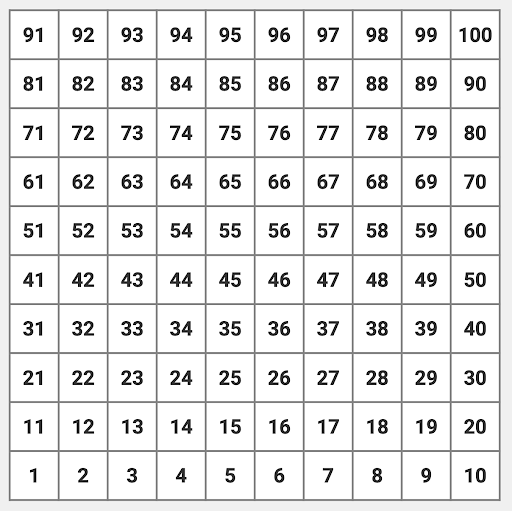Number grids are a great way for students to start looking for patterns in addition.  It begins to build an understanding of place value as students begin looking at what they notice about the tens and ones places.  Number grids are uniquely set up in rows of ten to model our base ten number system.  As students move across a row, they should notice the digit in the tens place stays the same until the end of the row and the ones place increases by one.  As they move up or down a row, they should notice that the ones place stays the same and the digit in the tens place either increases or decreases by one.

Please note the difference in these two number grid.  One grid goes from 0 to 110 top to bottom while the other goes from 1 to 100 bottom to top.  The one on the left is more commonly used in the classroom, however the one on the right more accurately matched a student's actions of addition or subtraction.  As students counts up in addition, the numbers go up the chart.  As a student counts back in subtraction, the numbers go down the chart.

#####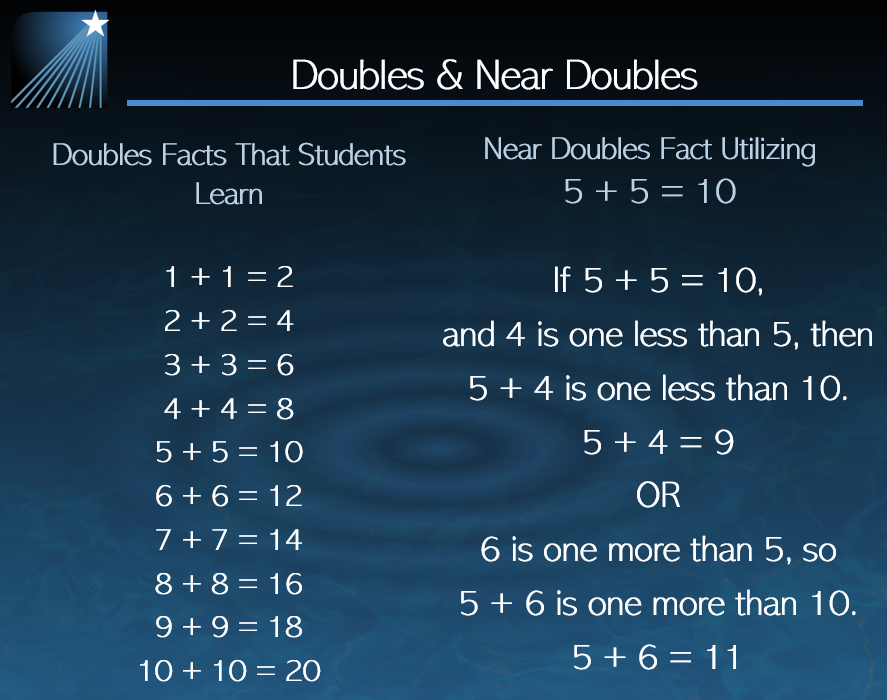The strategy of doubles and near doubles utilizes a students understanding of halving and doubling quantities.  It then builds on the known doubles facts to find near doubles (doubles plus one or doubles minus one).

For example, if a student knows the fact of 7 + 7 = 14, they can use that fact to support adding:

•   6 + 7
•   7 + 6
•   8 + 7
•   7 + 8

#### 7 + (7 + 1) = 14 + 1 or 7 + 8 = 15

Base Ten Blocks

Base ten blocks are a tool utilized many ways in mathematics.  In this case, we will be using the base ten blocks representing hundreds, tens, and ones to show the action of joining groups.  Students should start with building representations of each number, then join the sets to make the sum.  As students learn this strategy, start with numbers where regrouping is not needed.  As students become familiar with the action of joining the groups, begin the discussions of individual digits in a place value, explore what students think you could do about having more than nine of one particular place value.  Students can then model making fair trades.  As students become more comfortable with the physical manipulatives, move into the visual representation or sketch of the base ten blocks.  Teach students that they do not have to draw out individual units, but use "shorthand" sketches of the base ten blocks (a square for the hundreds, a line for the tens, and a dot for the ones).  Students can then relate their models to the more abstract standard algorithm for addition.  This progression of learning is modeled in the video.

##### Open Number Line

We are all familiar with the number line that young children use.  Typically it begins at zero with and counts up by one.  An open number line is a blank number line that can be used for any values along the number line that would be useful in solving problems.  Typically tick marks are not included on the open number line and as number are added to the number line, they may not be to scale.  The open number line is useful in making a representation to record steps of mental computation.  Watch the video to see an example of how to use open number lines with addition.

##### Compensation

Compensation is a strategy, often carried out in mental math, where one or more numbers is adjusted to make easier-to-use numbers for mental math.  For example some value may be taken from one addend and given to the other addend to make the problem easier to solve.  In the problem 59 + 32, 59 is only one away from 60 and 60 would be easier to add to than the 59, so taking one away from 32 (leaving behind 31) and giving it to the 59 (making 60), gives us the problem 60 + 31.  The problems 59 + 32 and 60 + 31 are equivalent, but 60 + 31 is something that most could consider easier to solve mentally.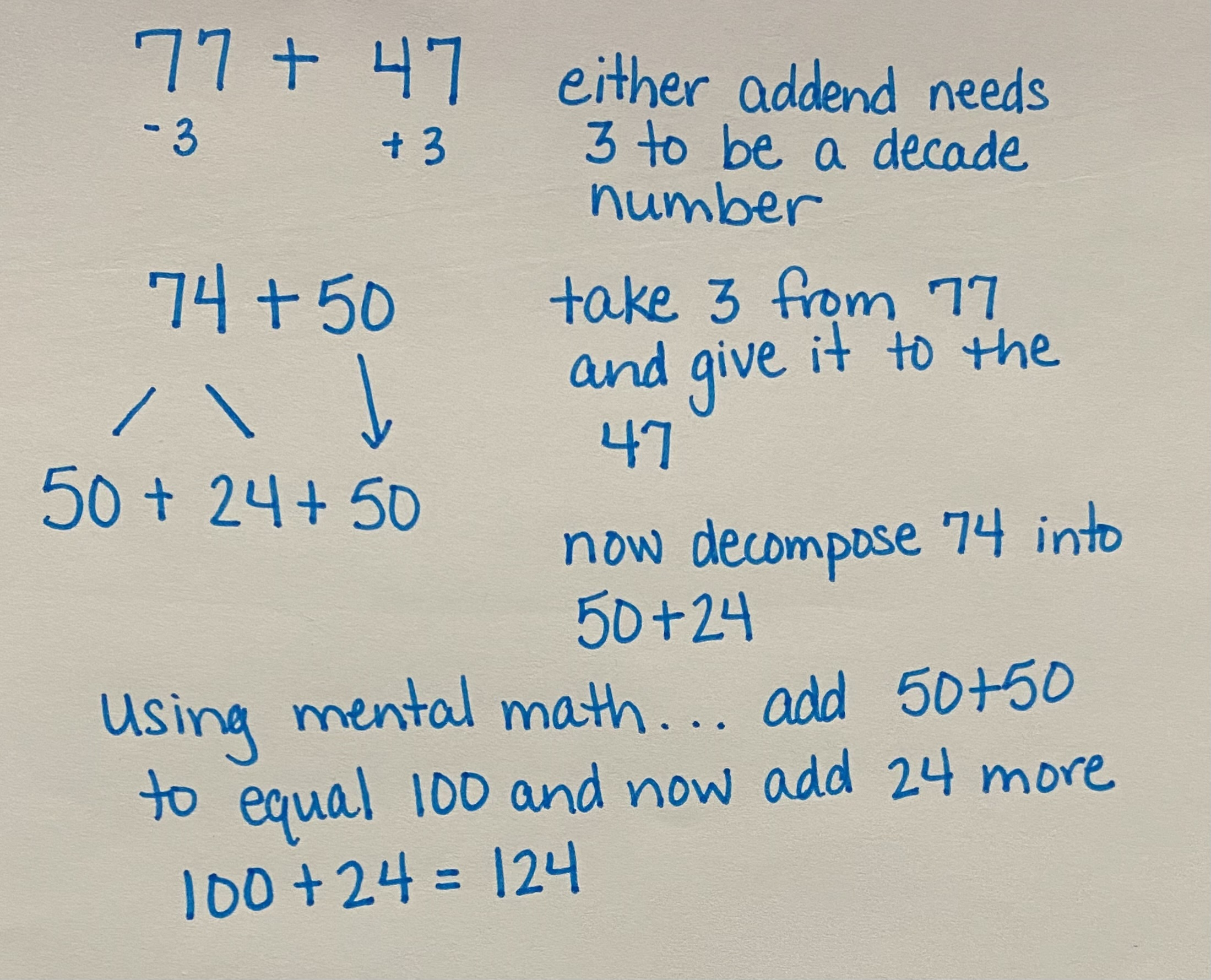Compensation decomposes (takes apart) or recomposes (puts back together) numbers to make addition easier to solve mentally.  Students need to understand how numbers can be broken apart and put back together to strengthen their number sense skills.

##### Making "Friendly" Numbers

Making "friendly" numbers refers to changing a number in a problem to one that may be easier to use for written or mental math.  These numbers may include landmark (like 25, 50, 75, etc.) or decade numbers (like 10, 20, 30, 40, 50, etc.).  "Friendly" numbers may be used in other strategies such as compensation and open number lines.  This strategy can also be used on its own, by adding to or subtracting from one of the addends and then removing or adding back in the amount to the sum.  For example, when solving 36 + 56, 36 is 4 away from 40, so by adding on 4 to the addend, means that 4 would need to be removed from the sum.  By adding 4 to the 36 and using 40 + 56 = 96, then removing the 4 that were added, 96 - 4 = 92, so 36 + 56 = 92.  It is important to keep in mind that the type of problem or the numbers used in the problem can lead to different strategies and the bigger concept is around number sense.

##### Partial Sums

The partial sums strategy utilizes place value to add each place value individually then bringing the sums within each place value together to make a final sum.

##### US Standard Algorithm

The US standard algorithm for addition is the strategy most adults think of when asked to add numbers.  Historically, it is a set of procedures that we were taught in our own schooling.  It is an efficient strategy, but it is strictly procedural.  When students can utilize more conceptual strategies to build their understanding of addition, they then can connect the procedures to that conceptual understanding.  Mastery of the standard algorithm is expected by the end of grade 4, however students will be introduced to this strategy much earlier as they are connecting strategies to build deeper knowledge.

#### Graham Fletcher Video - Progression of Addition and Subtraction